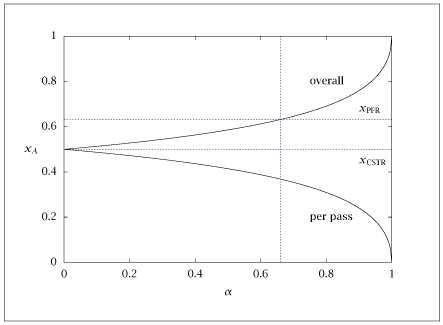## Figure 4.27:

### Overall and per-pass conversion of A as a function of fractional recycle, \alpha .## Code for Figure 4.27

### main.m


1
2
3
4
5
6
7
8
9
10
11
12
13
14
15
16
17
18
19
20
21
22
23
24
25
26
27
28
29
30
31
32
33
34
35
36
37
38
39
40
41
42
43
44
45
46
47
48
49
50
51
52
53
54
55
56
57
58
59
60
61
62
63
64
65
66
67
68
69
70
71
72
73
74
75
76
77
78
79
80
81
82
83
84
85
86
87
88
89
90
91
92
93% Copyright (C) 2001, James B. Rawlings and John G. Ekerdt
%
% This program is free software; you can redistribute it and/or
% modify it under the terms of the GNU General Public License as
% published by the Free Software Foundation; either version 2, or (at
% your option) any later version.
%
% This program is distributed in the hope that it will be useful, but
% WITHOUT ANY WARRANTY; without even the implied warranty of
% MERCHANTABILITY or FITNESS FOR A PARTICULAR PURPOSE.  See the GNU
% General Public License for more details.
%
% You should have received a copy of the GNU General Public License
% along with this program; see the file COPYING.  If not, write to
% the Free Software Foundation, 59 Temple Place - Suite 330, Boston,
% MA 02111-1307, USA.

%
% Find the separator, 2-cstr arrangement taht achieves the same outlet
% concentration as a single pfr.
% Marty Feinberg mentioned result that you need (s+1) cstrs in which s
% is the number of linearly independent reactions to achieve the pfr
% concentraion vector
%
% A -- > B, r=kc_A
%
% 11/8/99
%
% Revised 7/24/2018

k = 1;
theta = 1;
ktheta = k*theta;
xpfr = 1 - exp(-ktheta);
xcstr = ktheta/(1 + ktheta);

% compute the overall conversion (Na3) for various alpha fraction
% values of recycle, ntype = 1

p = struct(); %Create structure to pass parameters to fsolve function
p.ntype = 1;
p.ktheta = ktheta;
p.Na3 = NaN; %Don't need Na3 passed in for ntype = 1

nalpha = 250;
xalpha = linspace(0,1,nalpha)';
x = [1; 1/(1 + ktheta); 1/(1 + ktheta)];
xrec = zeros (nalpha,1);
xpass = zeros (nalpha,1);

for i = 1: nalpha
p.alpha = xalpha(i);
x0 = x;
[x, fval, info] = fsolve(@(x) recycle_reactor(x,p),x0);
Na1 = x(1);
Na2 = x(2);
Na3 = x(3);
xrec(i) = 1 - Na3;
xpass(i) = (Na1 - Na2)/Na1;
end

%plot(xalpha,xrec)
table = [xalpha xrec xpass];
%save -ascii pfr_sep_cstr.dat table;

% find the alpha recycle fraction for the PFR overall conversion,
% ntype=2

p.ntype = 2;
pata.ktheta = ktheta;
p.alpha = NaN; % Don't need alpha passed in for ntype = 2
p.Na3 = 1 - xpfr;

x = [1; 1/(1 + ktheta); 0];
x0 = x;
[x, fval, info] = fsolve(@(x) recycle_reactor(x,p),x0);
Na1   = x(1);
Na2   = x(2);
alpha = x(3);
auxtable = [0 xpfr xcstr alpha 0;
1 xpfr xcstr alpha 1];

%save -ascii pfr_sep_cstr_aux.dat auxtable;
save pfr_sep_cstr.dat table auxtable

if (~ strcmp (getenv ('OMIT_PLOTS'), 'true')) % PLOTTING
plot (table(:,1),table(:,2:3),...
auxtable(:,1),auxtable(:,2:3),...
auxtable(:,4),auxtable(:,5));
% TITLE
end % PLOTTING



### recycle_reactor.m


1
2
3
4
5
6
7
8
9
10
11
12
13
14
15
16
17
18
19
20function resid = recycle_reactor(x,p)
Na1 = x(1);
Na2 = x(2);
if (p.ntype == 1)
Na3 = x(3);
alpha = p.alpha;
ktheta = p.ktheta;
elseif (p.ntype == 2)
Na3 = p.Na3;
alpha = x(3);
ktheta = p.ktheta;
else
error ('recycle_reactor: ntype out of range')
end%if
% reactor balance
% feed mixer
% outlet splitter
resid = [(1 + ktheta/Na1)*Na2 - Na1;
alpha*Na2 + 1 - Na1;
Na3 - (1 - alpha)*Na2];